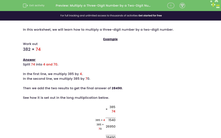# Multiply a Three-Digit Number by a Two-Digit Number

In this worksheet, students will use long multiplication to find the product of a three-digit number and a two-digit number.Key stage:  KS 2

Curriculum topic:   Number: Addition, Subtraction, Multiplication and Division

Curriculum subtopic:   Multiply to Four Digits (Long Multiplication)

Popular topics:   Numbers worksheets

Difficulty level:#### Worksheet Overview

In this activity, we will be multiplying three-digit numbers by two-digit numbers.

Example

Work out

382 × 74

Split 74 into 4 and 70.

In the first line, we multiply 385 by 4.

In the second line, we multiply 385 by 70.

Then we add the two results to get the final answer of 28,490.

See how it is set out in the long multiplication below.

 × 385 74 385 × 4 1540 385 × 70 26950 28490

Let's have a go at some questions now.### What is EdPlace?

We're your National Curriculum aligned online education content provider helping each child succeed in English, maths and science from year 1 to GCSE. With an EdPlace account you’ll be able to track and measure progress, helping each child achieve their best. We build confidence and attainment by personalising each child’s learning at a level that suits them.

Get started